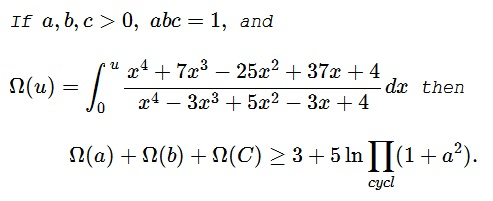# An Inequality with Integrals

### Problem### Solution 1

\displaystyle \begin{align} \Omega(u)&=\int_0^u\frac{x^4+7x^3-25x^2+37x+4}{x^4-3x^3+5x^2-3x+4}dx\\ &=\int_0^u\frac{(x^4-3x^3+5x^2-3x+4)+(10x^3-30x^2+40x)}{x^4-3x^3+5x^2-3x+4}dx\\ &=\int_0^u\left(1+\frac{10x(x^2-3x+4)}{(x^2+1)(x^2-3x+4)}\right)dx\\ &=\int_0^u\left(1+\frac{10x}{x^2+1}\right)dx\\ &=u+5\ln(u^2+1). \end{align}

It follows that, by the AM-GM inequality, that

\displaystyle \begin{align} \Omega(a)+\Omega(b)+\Omega(C)&\ge (a+b+c)+5\sum_{cycl}\ln(a^2+1)\\ \Omega(a)+\Omega(b)+\Omega(C)&\ge 3\sqrt{abc}+5\ln\prod_{cycl}(a^2+1)\\ &=3+5\ln\prod_{cycl}(a^2+1). \end{align}

### Solution 2

$\displaystyle \Omega (u):=\int_0^u f(x)\, dx$ where

$\displaystyle f(x)=\frac{x^4+7 x^3-25 x^2+37 x+4}{x^4-3 x^3+5 x^2-3 x+4}.$

Simplifying we get $\displaystyle f(x)=\frac{10 x}{x^2+1}+1.$

We have the antiderivative as

$\displaystyle \int f(x)\, dx= 5 \log \left(x^2+1\right)+x.$

$\displaystyle\sum_{cycl}(\Omega)=\sum_{cycl}a+ 5\log \left(x^2+1\right).$ Since $a+b+c \geq 3 \sqrt{a b c}=3$, by AM-GM, we get the required proof.

### Acknowledgment

The problem was kindly posted at the CutTheKnotMath facebook page by Dan Sitaru. The problem is by Dan and was previously published in the Romanian Mathematical Magazine. Solution 1 is by Seyran Ibrahimov; Solution 2 is by N. N. Taleb.

### Inequalities with the Product of Variables as a Constraint